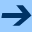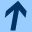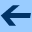The number of invariant subspaces under a linear operator on finite vector spaces

## The number of invariant subspaces under a linear operator on finite vector spaces

Advances in Mathematics of Communications 5, No. 2, 407-416, 2011. Proceedings of ALGEBRAIC COMBINATORICS AND APPLICATIONS (ALCOMA10). DOI 10.3934/amc.2011.5.407

Abstract: Let V be an n-dimensional vector space over the finite field 𝔽q and T a linear operator on V. For each k∈ {1,…,n} we determine the number of k-dimensional T-invariant subspaces of V. Finally, this method is applied for the enumeration of all monomially nonisometric linear (n,k)-codes over 𝔽q.

harald.fripertinger "at" uni-graz.at, October 10, 2019